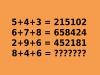BRAIN TEASERSBrain Teasers User Profile

# Rositsa Tsvetkova

rank
842
points
1
See full ranking list
short ranking list
 840 Peter Jansson 1 841 Eeda Gunavardhan 1 842 Rositsa Tsvetkova 1 843 Kritz Shan 1 844 Sky Donsila 1
 Mathematical Puzzle: IF 5+4+... Mathematical Puzzle: IF 5+4+3=215102, 6+7+8=658424 and 2+9+6=452181 THEN 8+4+6=?